Successfully reported this slideshow.
We use your LinkedIn profile and activity data to personalize ads and to show you more relevant ads. You can change your ad preferences anytime.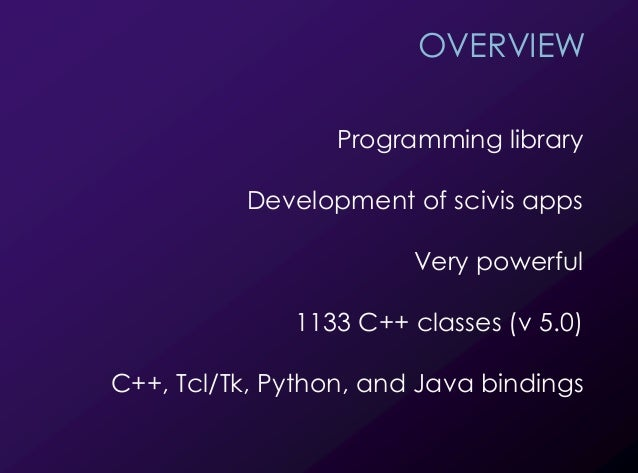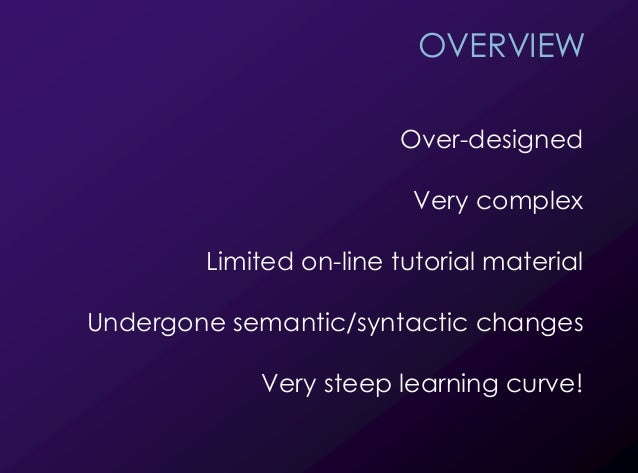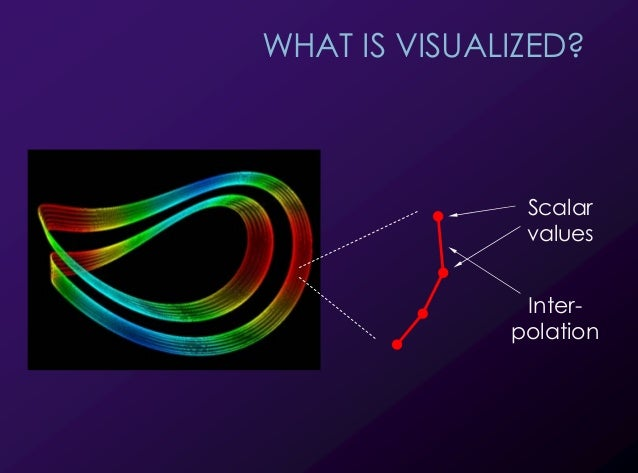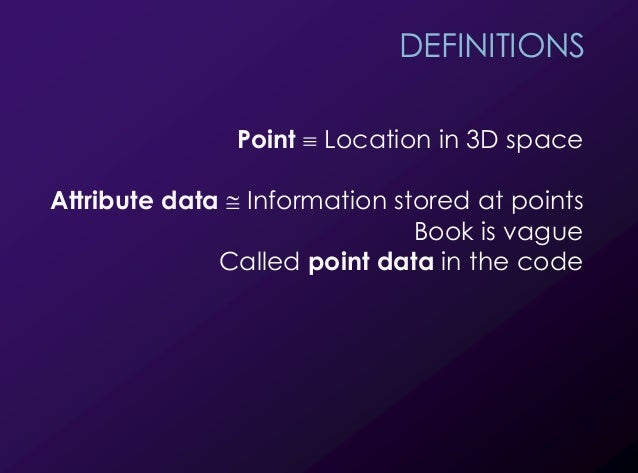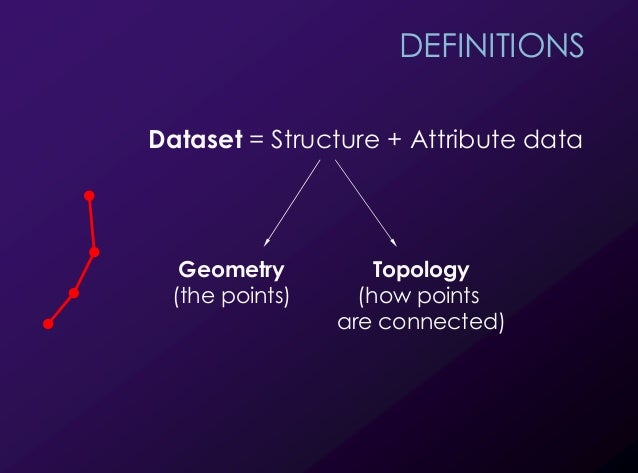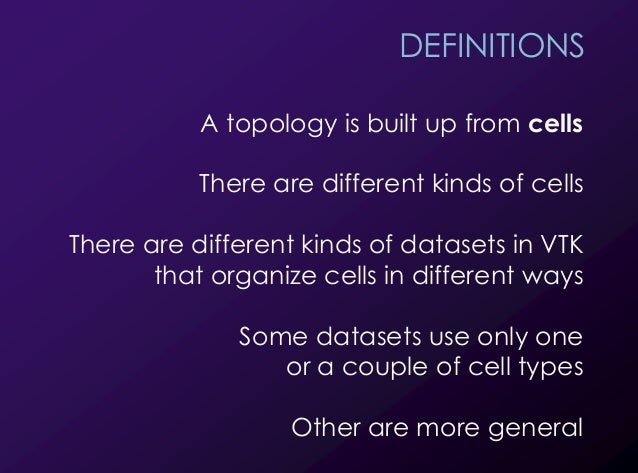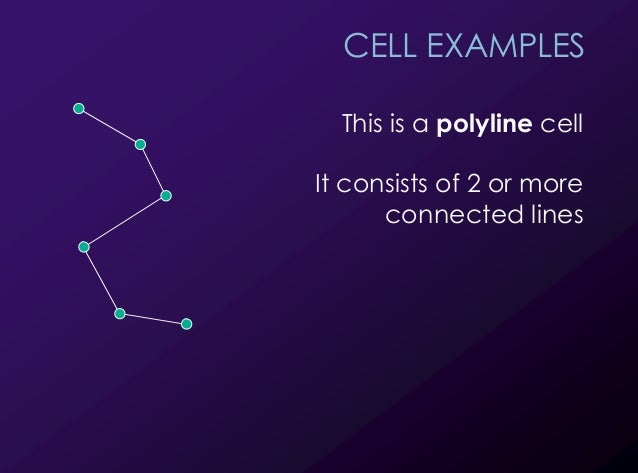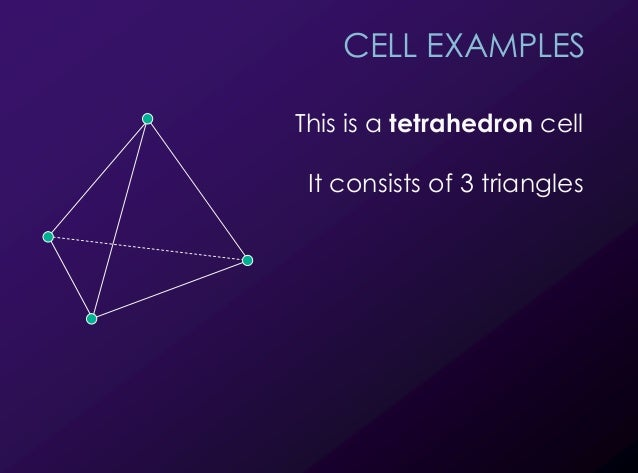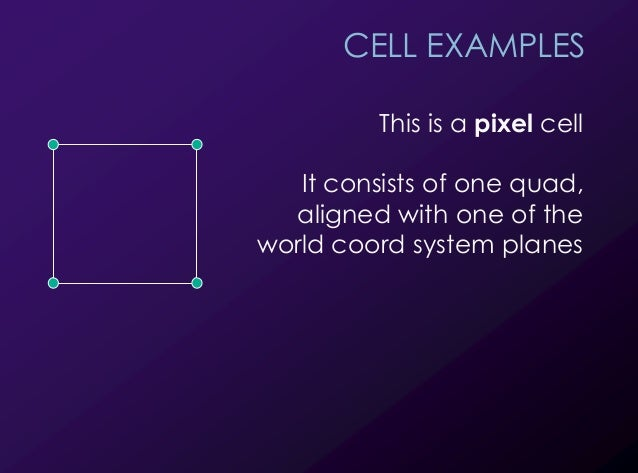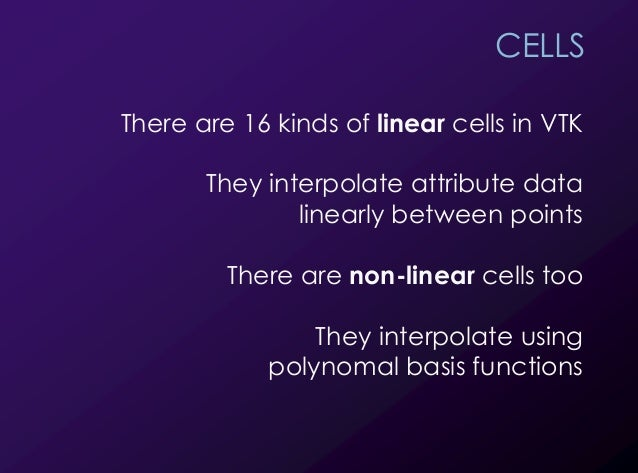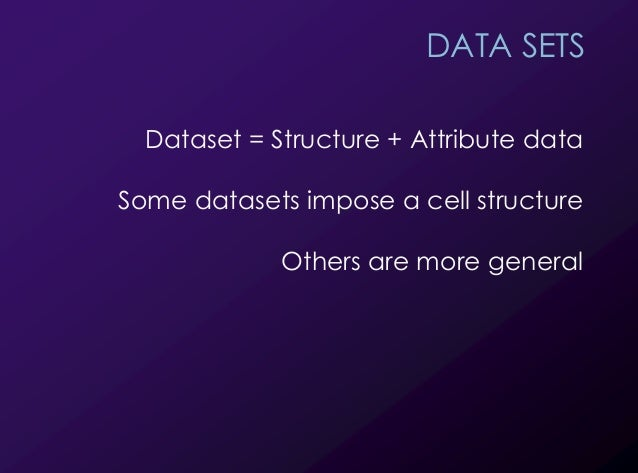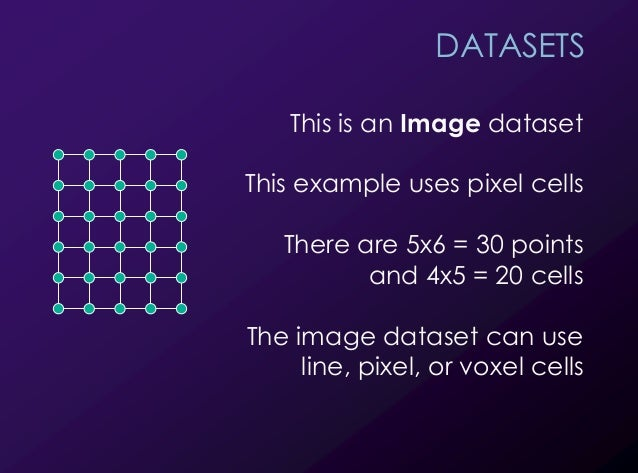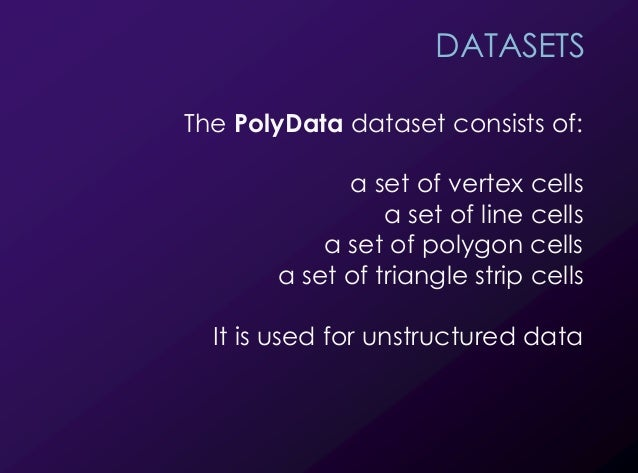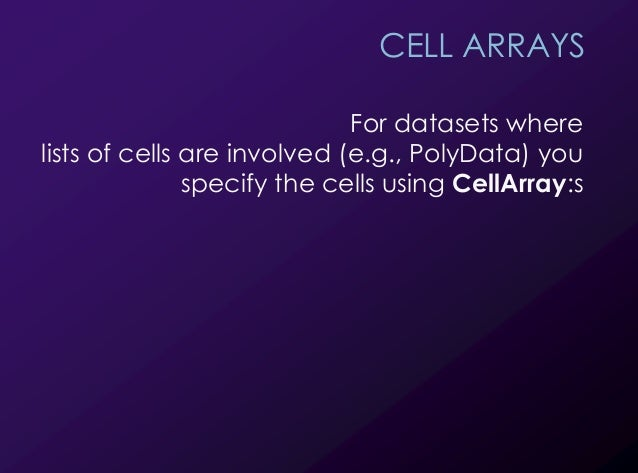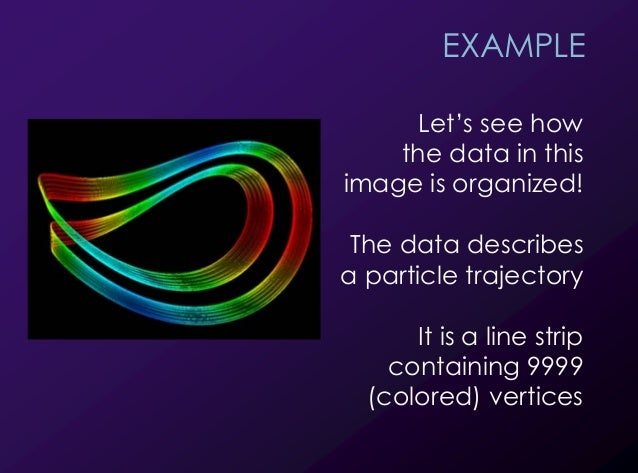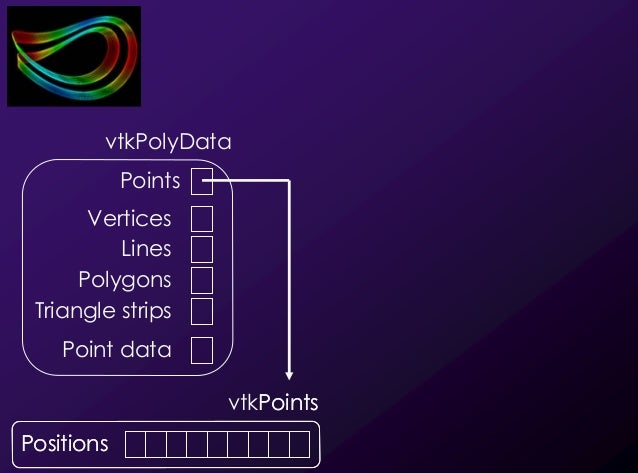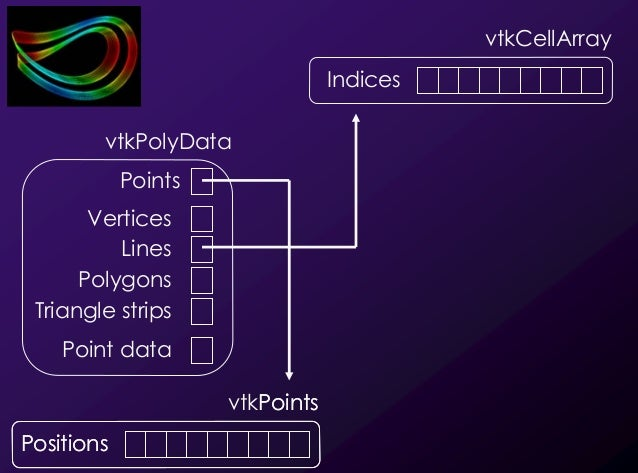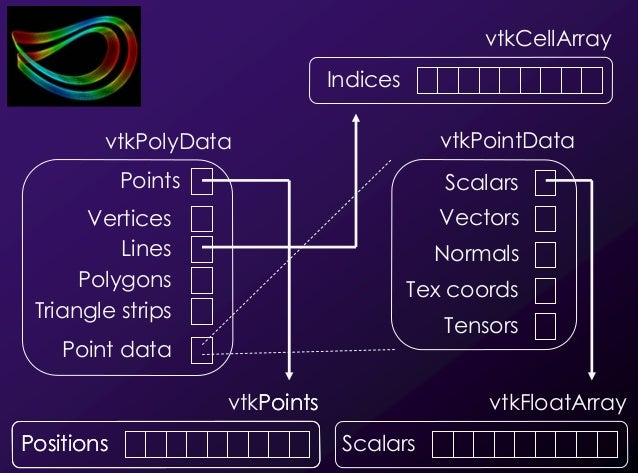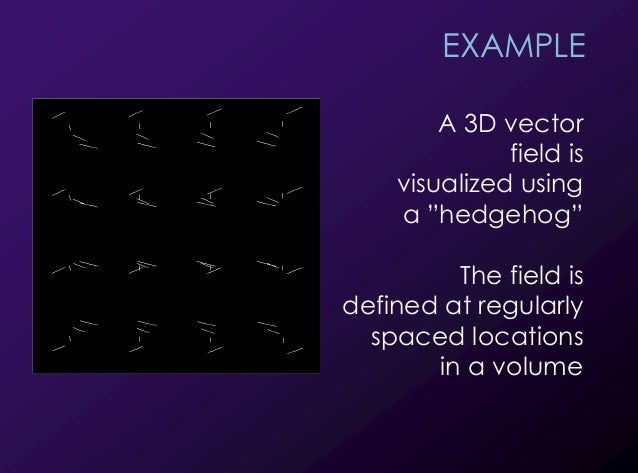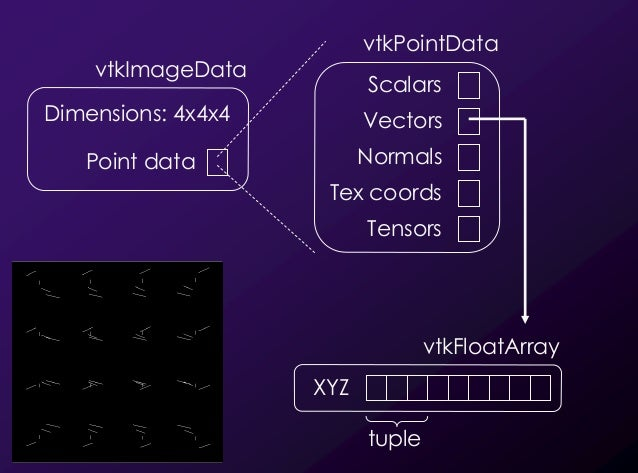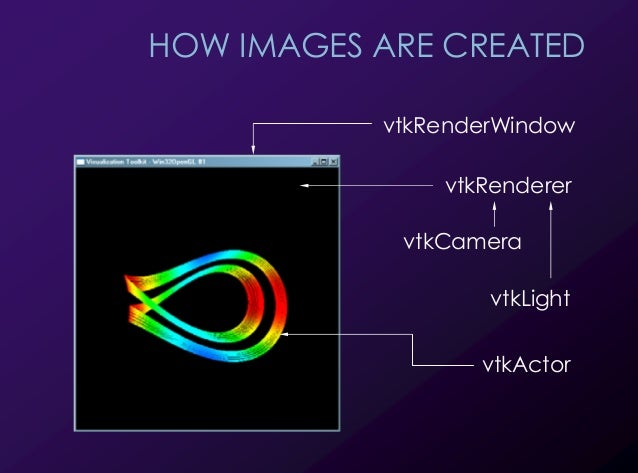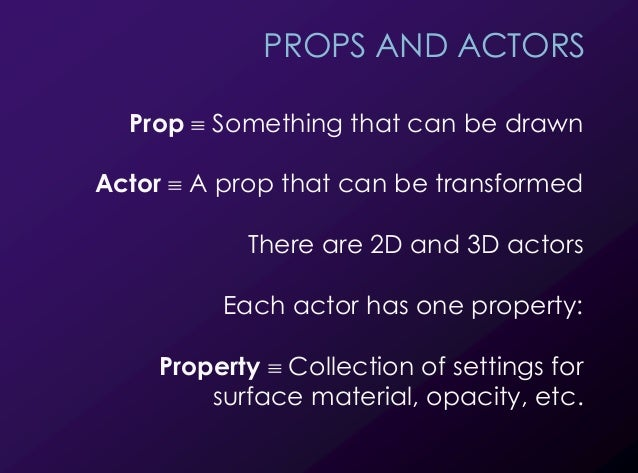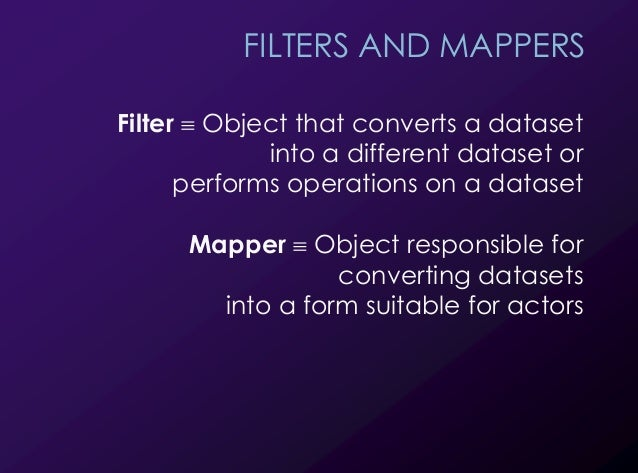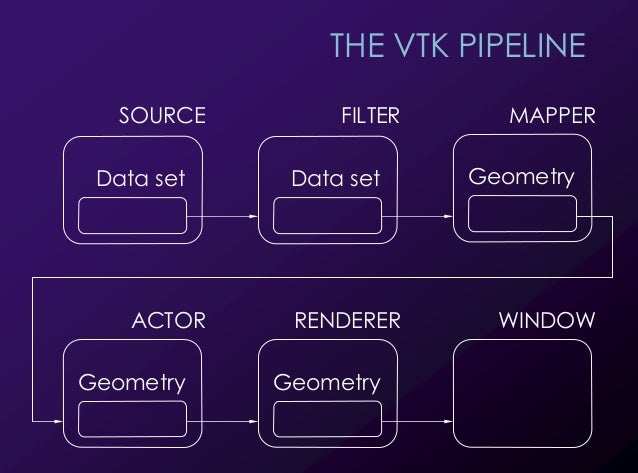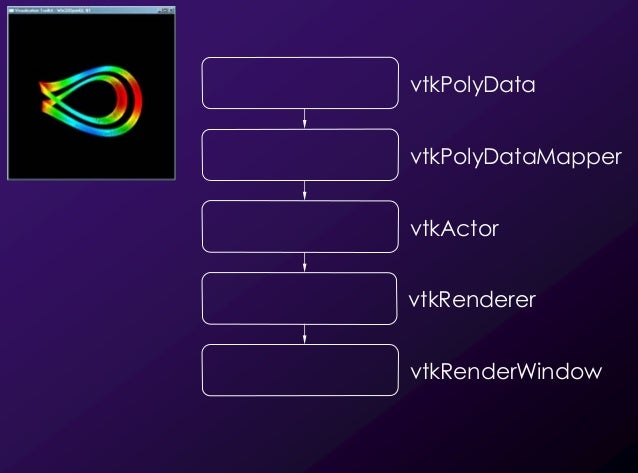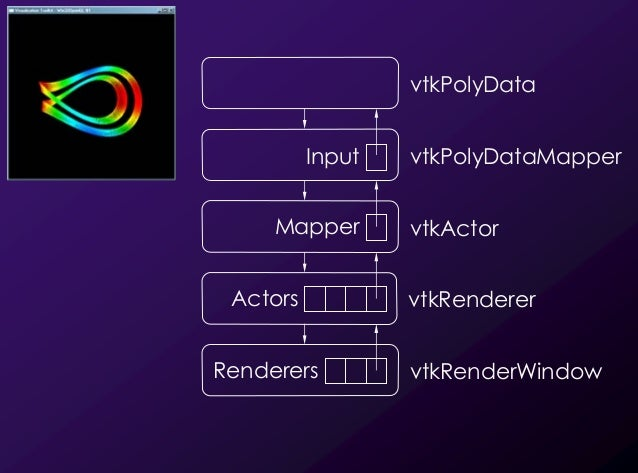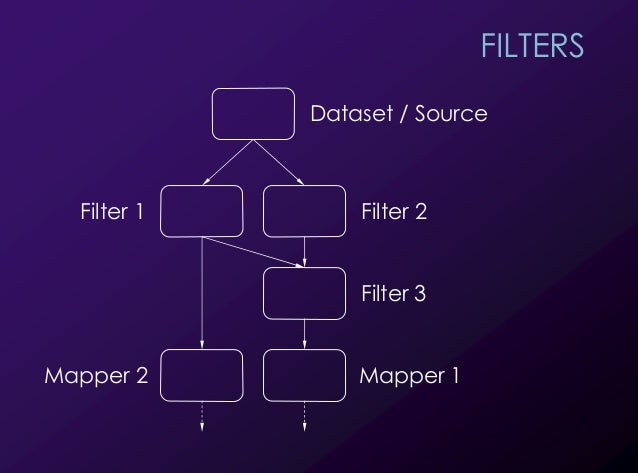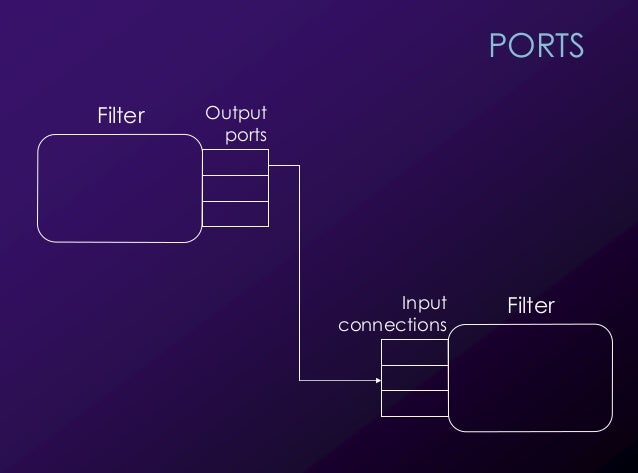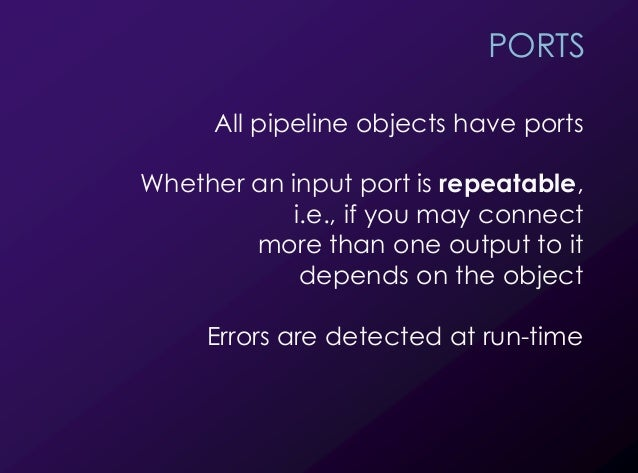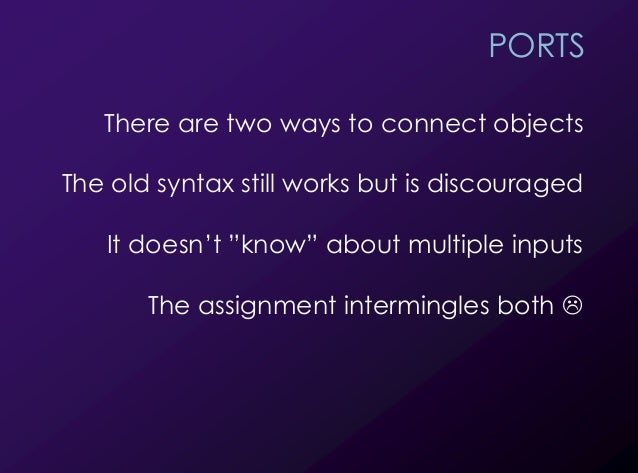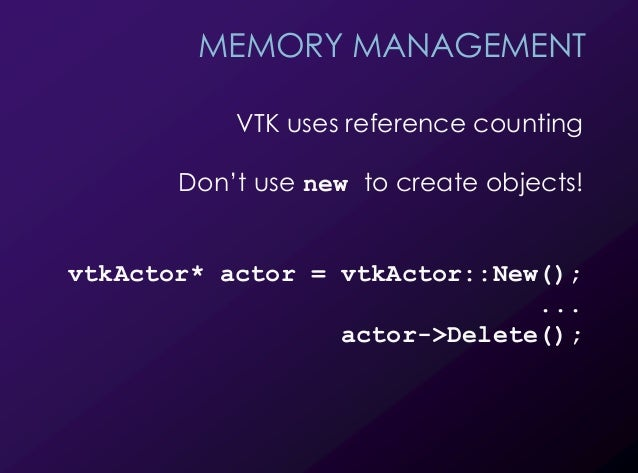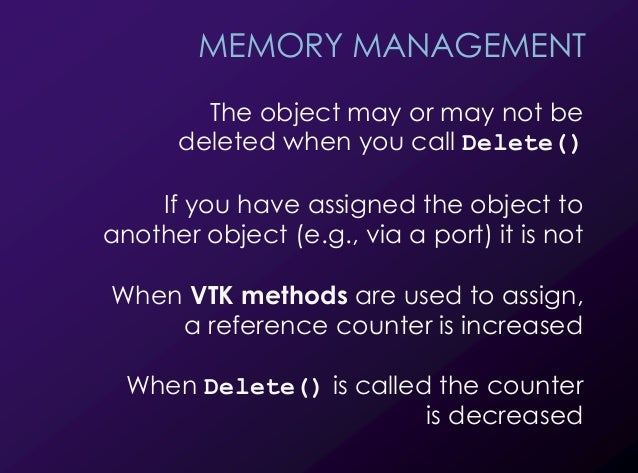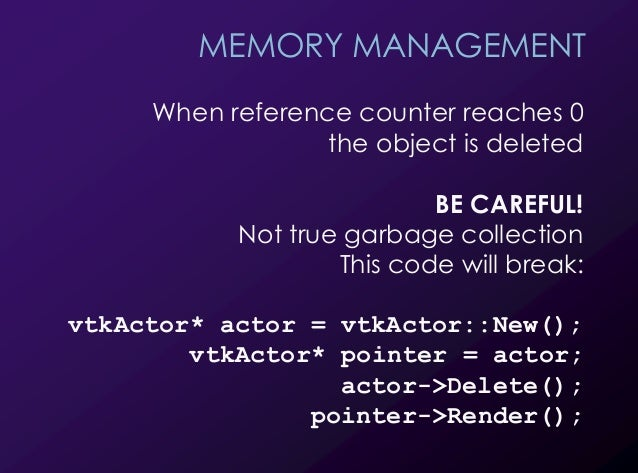Upcoming SlideShare
Loading in …5
×

# Introduction to VTK

Presentation by Gustav Taxén, Ph.D

Original version can be found at http://www.csc.kth.se/utbildning/kth/kurser/DD2257/visual09/VTK-090325-GT.pdf

• Full Name
Comment goes here.

Are you sure you want to Yes No
Your message goes here• Be the first to comment

### Introduction to VTK

1. 1. 1 INTRODUCTION TO VTK Gustav Taxén, Ph. D. Associate Professor School of Computing Science and Communication Royal Institute of Technology, Stockholm gustavt@csc.kth.se
2. 2. 2 OVERVIEW Programming library Development of scivis apps Very powerful 1133 C++ classes (v 5.0) C++, Tcl/Tk, Python, and Java bindings
3. 3. 3 OVERVIEW Over-designed Very complex Limited on-line tutorial material Undergone semantic/syntactic changes Very steep learning curve!
4. 4. 4 WHAT IS VISUALIZED? Scalar values Inter- polation
5. 5. 5 DEFINITIONS Point ! Location in 3D space Attribute data " Information stored at points Book is vague Called point data in the code
6. 6. 6 DEFINITIONS Dataset = Structure + Attribute data Geometry (the points) Topology (how points are connected)
7. 7. 7 DEFINITIONS A topology is built up from cells There are different kinds of cells There are different kinds of datasets in VTK that organize cells in different ways Some datasets use only one or a couple of cell types Other are more general
8. 8. 8 CELL EXAMPLES This is a polyline cell It consists of 2 or more connected lines
9. 9. 9 CELL EXAMPLES This is a tetrahedron cell It consists of 3 triangles
10. 10. 10 CELL EXAMPLES This is a pixel cell It consists of one quad, aligned with one of the world coord system planes
11. 11. 11 CELLS There are 16 kinds of linear cells in VTK They interpolate attribute data linearly between points There are non-linear cells too They interpolate using polynomal basis functions
12. 12. 12 DATA SETS Dataset = Structure + Attribute data Some datasets impose a cell structure Others are more general
13. 13. 13 DATASETS This is an Image dataset This example uses pixel cells There are 5x6 = 30 points and 4x5 = 20 cells The image dataset can use line, pixel, or voxel cells
14. 14. 14 DATASETS The PolyData dataset consists of: a set of vertex cells a set of line cells a set of polygon cells a set of triangle strip cells It is used for unstructured data
15. 15. 15 CELL ARRAYS For datasets where lists of cells are involved (e.g., PolyData) you specify the cells using CellArray:s
16. 16. 16 EXAMPLE Let’s see how the data in this image is organized! The data describes a particle trajectory It is a line strip containing 9999 (colored) vertices
17. 17. 17 Points Positions vtkPoints Positions vtkPolyData Lines Points Point data Polygons Vertices Triangle strips We use a PolyData dataset. The geometry of the dataset are the locations of the vertices. They are specified as VTK points. A point always has 3 dimensions (x, y, z).
18. 18. 18 vtkCellArray Indices Points Positions vtkPoints Positions vtkPolyData Lines Points Point data Polygons Vertices Triangle strips Since this DataSet doesn’t impose a cell structure, we need to specifiy the topology explicitly, i.e., the cells of the dataset, i.e., what the relationship between the points is. THIS IMAGE IS VERY IMPORTANT – EVERYONE SHOULD UNDERSTAND IT BEFORE MOVING ON! A PolyData dataset can connect points in different ways. It can contain vertices, lines, polygons, and/or triangle strips. Since PolyData is an unstructured dataset, we need to specify cells explicitly. It must be done via CellArrays. There is one CellArray for the vertices, one for the lines, one for the polygons, and one for the tristrips. A CellArray is an integer-valued array containing point list indices. Together, these indices specify one or more cells. How the indices are combined into cells are determined by whether we assign the CellArray as the vertices, the lines, the polygons, or the trianglestrips of the dataset. The format of the CellArray is as follows: The first value is the number of indices for the first cell. Then follows the indices themselves. The next value is the number of indices for the second cell. Then follows the indices for the second cell, and so on. In our case, we choose to use ONE cell. This cell will contain 9999 indices - one for each point. If a line contains more than two indices it is automatically interpreted as a line strip. Since the data points are in order, the indices first index is 0, the second is 1, and so on up to 9998.
19. 19. 19 vtkCellArray Indices Points Positions vtkPoints Positions vtkFloatArray Scalars vtkPolyData Lines Points Point data Polygons Vertices Triangle strips vtkPointData Scalars Vectors Normals Tex coords Tensors The next step is to assign the scalar values to the points. The dataset contains ”attribute data”, which is VTK speak for ”values defined at point locations”. These values are the ones that are interpolated by VTK when we generate the image. In the API, the ”attribute data” is denoted as ”point data”. At each point, we can store a scalar, a 3D vector, a normal, a 2D tex coord, or a tensor. I’m not sure whether it’s OK to store more than one of these at each point. Here, we want to store scalars, which means that we need one floating-point value for each geometry point. This has been provided to us in the same data file that contains the particle trajectory data. ”Attribute data” (or ”Point data”) is stored in FloatArrays. If the data is multidimensional, it is interleaved in the array. For example, a 3D vector at each point would be stored as X0 Y0 Z0 X1 Y1 Z1, and so on. The point data is ”owned” by the polydata object. It has ”slots” for scalars, vectors, normals, etc. We can either use a pointer to a FloatArray in these slots, or we can choose to copy the data into the PointData object.
20. 20. 20 EXAMPLE A 3D vector field is visualized using a ”hedgehog” The field is defined at regularly spaced locations in a volume
21. 21. 21 vtkImageData Point data vtkFloatArray XYZ Dimensions: 4x4x4 vtkPointData Scalars Vectors Normals Tex coords Tensors tuple Since the data is regularly spaced, we can use a ImageData dataset. In VTK, ImageData can be both 2D and 3D, as long as it is regular and is aligned with the global coord axes. We specify that the number of samples in the dataset along each axis is 4x4x4. We then set a world-space spacing between each sample. This completely defines both the geometry and the topology. What remains to be done is to assign the ”attribute data” (or ”point data”) at each point. Again, the 3D vectors to be assigned to the points are stored in a FloatArray. Here, we need 3 floating-point values per geometry point. These 3 values are referred to as a tuple. All datasets in VTK use the vtkPointData class to assign attribute data to points. In this case, we want to store a vector at each point, so we use the ”vector” slot of the PointData class.
22. 22. 22 HOW IMAGES ARE CREATED vtkRenderWindow vtkRenderer vtkCamera vtkLight vtkActor
23. 23. 23 PROPS AND ACTORS Prop ! Something that can be drawn Actor ! A prop that can be transformed There are 2D and 3D actors Each actor has one property: Property ! Collection of settings for surface material, opacity, etc.
24. 24. 24 FILTERS AND MAPPERS Filter ! Object that converts a dataset into a different dataset or performs operations on a dataset Mapper ! Object responsible for converting datasets into a form suitable for actors
25. 25. 25 THE VTK PIPELINE Data set SOURCE MAPPER Data set FILTER Geometry ACTOR Geometry RENDERER Geometry WINDOW
26. 26. 26 vtkPolyData vtkPolyDataMapper vtkActor vtkRenderer vtkRenderWindow
27. 27. 27 vtkPolyData vtkPolyDataMapper vtkActor vtkRenderer vtkRenderWindow Input Mapper Actors Renderers
28. 28. 28 FILTERS Dataset / Source Filter 1 Filter 2 Filter 3 Mapper 1Mapper 2
29. 29. 29 PORTS Filter Output ports FilterInput connections
30. 30. 30 PORTS All pipeline objects have ports Whether an input port is repeatable, i.e., if you may connect more than one output to it depends on the object Errors are detected at run-time
31. 31. 31 PORTS There are two ways to connect objects The old syntax still works but is discouraged It doesn’t ”know” about multiple inputs The assignment intermingles both !
32. 32. 32 MEMORY MANAGEMENT VTK uses reference counting Don’t use new to create objects! vtkActor* actor = vtkActor::New(); ... actor->Delete();
33. 33. 33 MEMORY MANAGEMENT The object may or may not be deleted when you call Delete() If you have assigned the object to another object (e.g., via a port) it is not When VTK methods are used to assign, a reference counter is increased When Delete() is called the counter is decreased
34. 34. 34 MEMORY MANAGEMENT When reference counter reaches 0 the object is deleted BE CAREFUL! Not true garbage collection This code will break: vtkActor* actor = vtkActor::New(); vtkActor* pointer = actor; actor->Delete(); pointer->Render();